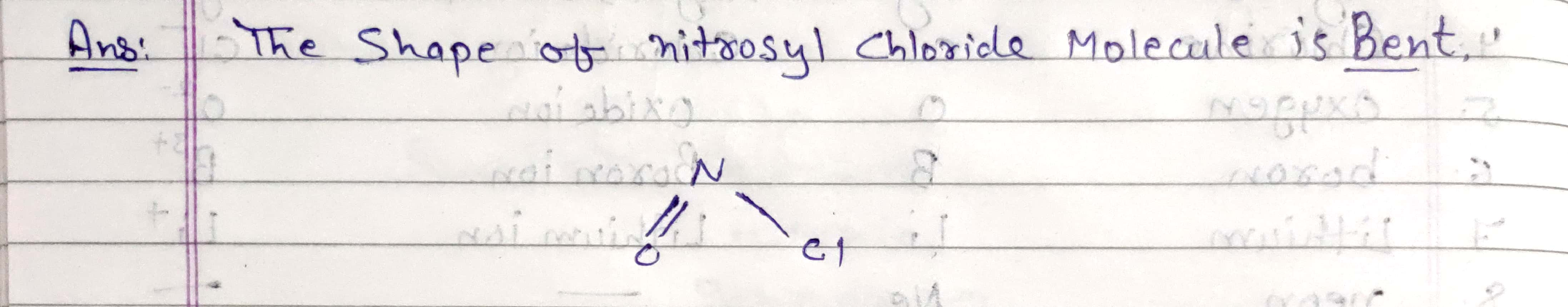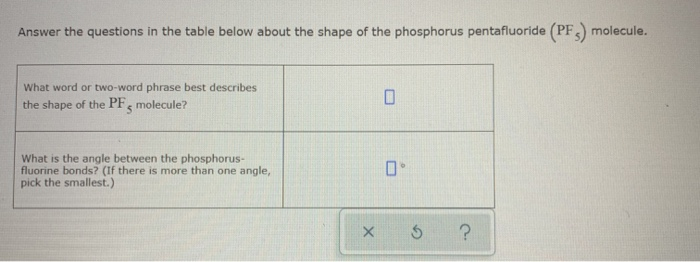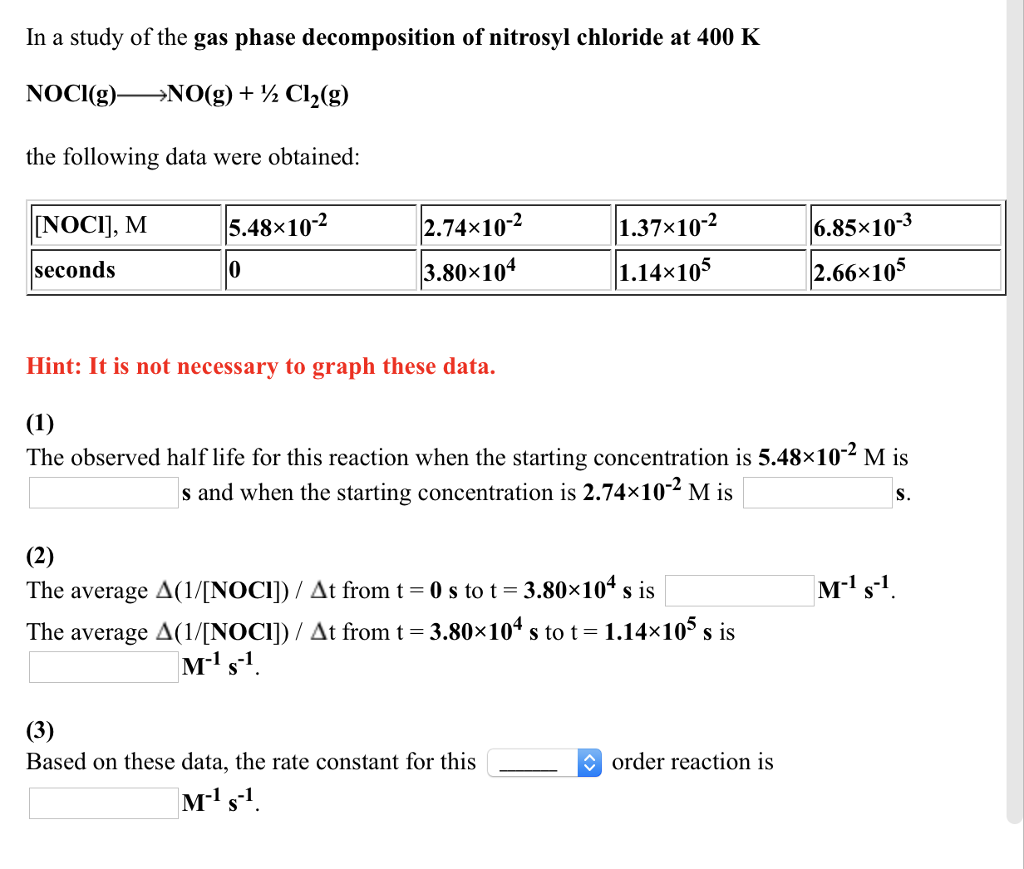Question

# What word or two-word phrase best describes the shape of the nitrosyl chloride (NOCl) molecule?

What word or two-word phrase best describes the shape of the nitrosyl chloride (NOCl) molecule?#### Earn Coins

Coins can be redeemed for fabulous gifts.

Similar Homework Help Questions
• ### What word or two-word phrase best describes the shape of the fluoroform ( CHF3 ) molecule?

What word or two-word phrase best describes the shape of the fluoroform ( CHF3 ) molecule?

• ### 1) Nitrosyl chloride (NOCl) decomposes at high temperature

1) Nitrosyl chloride (NOCl) decomposes at high temperature. The equation is 2 NOCl (g) -> 2 NO (g) + Cl2 (g) at 227oC Using delta Ho = 81.2 kJ and delta So = 128 J/K, calculate the value of the equilibrium constant for this reaction. I do not know how to start this problem or what formula I should use.

• ### Nitrosyl chloride (NOCl) decomposes at high temperature

Nitrosyl chloride (NOCl) decomposes at high temperature. The equation is 2 NOCl (g) -> 2 NO (g) + Cl2 (g) at 227C Using delta H = 81.2 kJ and delta S = 128 J/K, calculate the value of the equilibrium constant for this reaction. a 1.60 X 10-2 b. 2.1 X 10-7 c. 62.8 d. 4.9 X 106 e. 3,20 X 109 help no idea?

• ### Answer the questions in the table below about the shape of the phosphorus pentafluoride (PF3) molecule....Answer the questions in the table below about the shape of the phosphorus pentafluoride (PF3) molecule. What word or two-word phrase best describes the shape of the PF, molecule? What is the angle between the phosphorus- fluorine bonds? (If there is more than one angle, pick the smallest.) X 5 ?

• ### Nitrosyl chloride, NOCl, can be used as a nonaqueous solvent. It can undergo autodissociation with NO+...

Nitrosyl chloride, NOCl, can be used as a nonaqueous solvent. It can undergo autodissociation with NO+ and Cl– as the products. Identify which of the ions is a Lewis acid and which is a Lewis base, and explain why.

• ### 1) Nitrosyl chloride (NOCl) decomposes at high temperature

1) Nitrosyl chloride (NOCl) decomposes at high temperature. The equation is 2 NOCl (g) -> 2 NO (g) + Cl2 (g) at 227oC Using delta Ho = 81.2 kJ and delta So = 128 J/K, calculate the value of the equilibrium constant for this reaction. I do not know how to start this problem or what formula I should use. Chemistry(Please help) - DrBob222, Monday, April 23, 2012 at 7:50pm dGo = dHo - TdSo Then dGo = -RTlnK So...

• ### Draw three resonance structures for nitrosyl chloride (NOCl) and assign formal charges to each atom. (Nitrogen...

Draw three resonance structures for nitrosyl chloride (NOCl) and assign formal charges to each atom. (Nitrogen is the central atom) A) Which resonance structure is favored by formal charge considerations & why? B) For the favored resonance structure, what is the electron and molecular geometry around the nitrogen atom? What is the bond angle?

• ### The reaction between nitric oxide (NO) and chlorine (Cl2) to form nitrosyl chloride (NOCl) was found...

The reaction between nitric oxide (NO) and chlorine (Cl2) to form nitrosyl chloride (NOCl) was found to occur as an elementary reaction as follows: 2 NO (g) + Cl2 (g) à  2 NOCl (g) A table of the concentrations and initial rates data is shown below: [ NO ]0 (M)                 [ Cl2 ]0 (M)                  rate = - D[Cl2]/ Dt  (M / min) Run 1                    0.10                             0.10                                   0.18 Run 2                    0.10                             0.20                                   0.36 Run 3                    0.20                             0.20                                   1.44 The order for Cl2 in this reaction is: (a)  1                            (b)  45                         (c)  2                            (d)  180

• ### In a study of the gas phase decomposition of nitrosyl chloride at 400 K NOCl(g)-NO(g) +...In a study of the gas phase decomposition of nitrosyl chloride at 400 K NOCl(g)-NO(g) + ½ Cl2(g) the following data were obtained: [NOCI], M 5.48x10-2 2.74x10-2 1.37×10-2 6.85x10-3 seconds 3.80x104 1.14x10 2.66x105 Hint: It is not necessary to graph these data. The observed half life for this reaction when the starting concentration is 5.48×10-2 M is s and when the starting concentration is 2.74x10 M is S. Ms The average Δ(1/[NOCl) At from t-0 s to t-3.80x10" s is...

• ### If it takes 0.20 min to decompose 15% of a 0.300 M solution of nitrosyl chloride,what is k(the...

the decomposition of nitrosyl chloride is a second order reaction:NOCl(g)--->NO(g)+1/2Cl2(g)